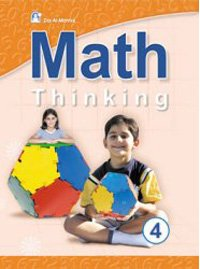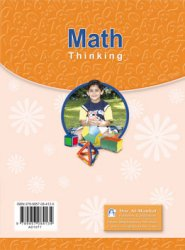+962 (6) 5533889
info@manhal.com
+962 (78) 7000522
support@manhal.com

# Math Thinking Level 04 book

• Book
• Description
• Related
• Rating
(0)
••5164
0
0
0
• Math Thinking 4 is one of Dar Al Manhal educational books that comes within Math Thinking curriculum which includes 6 books that teach children mathematics basics in English language.

Math Thinking 4 book is divided into twelve units which include numbers of 7 digits; millions, relations between them, and addition and subtraction within.

The book, Math Thinking 4, also teaches multiplication in two or three numbers, and multiplication in (10, 100, 1000), divisibility on (3 to 5), fractions, decimals , measurement, geometry, probability, and statistics.

The book, Math Thinking 4, also includes solving mathematical problems of each unit to test what children have learned.

152
Soft Cover
Product Dimensions and Weight
21 cm
28 cm
480 Gm
1124/5/2005
4
One
\$ 8.8
• Math
• Ammar Dkeidek And Others
• 2005
• English
• 9-11 Year
x
\$220